Chapter 2, Problem 1RCC### Single Variable Calculus: Early Tr...

8th Edition
James Stewart
ISBN: 9781305270343

#### Solutions

Chapter
Section### Single Variable Calculus: Early Tr...

8th Edition
James Stewart
ISBN: 9781305270343
Textbook Problem

# Explain what each of the following means and illustrate with sketch.(a) lim x → a f ( x ) = L (b) lim x → a + f ( x ) = L (c) lim x → a − f ( x ) = L (d) lim x → a f ( x ) = ∞ (e) lim x → ∞ f ( x ) = L

(a)

To determine

To explain: The meaning of limxaf(x)=L.

Explanation

Result used:

Definition of limit:

Let f(x) be a function is defined when x approaches to p then limxpf(x)=L, if for every number ε>0 there is some δ>0  such that |f(x)L|<ε whenever 0<|xp|<δ.

Graph: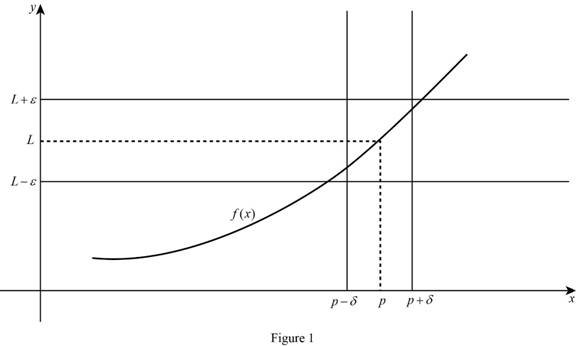Calculation:

The limit of the function limxaf(x)=L means the limit of f(x) equal to L when x approaches to a, if x is closer and closer to a from the both sides then the value of f(x) also closer and closer to L.

In the limit definition xa this means finding the limit of f(x) when x approaches to a, there no need to consider x=a.

There are three cases for define limxaf(x)=L.

Case (1):

The limit of the function limxaf(x)=L, if x approaches to a then the value of f(x) are closer to L and f(a) is L.

Graph: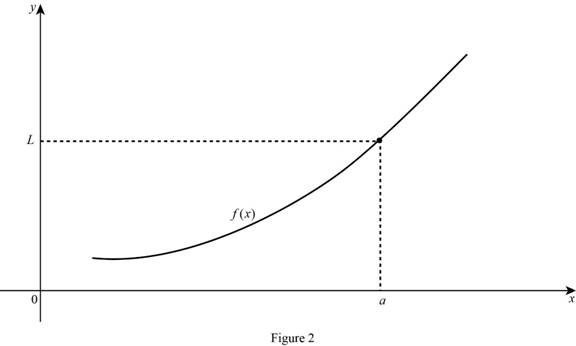Case (2):

The limit of the function limxaf(x)=L, if x approaches to a then the value of f(x) are closer to L and f(a) is undefined.

Case (3):

The limit of the function limxaf(x)=L, if x approaches to a then the value of f(x) are closer to other than L.

Graph: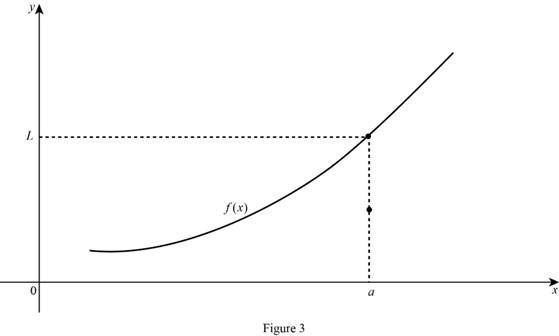(b)

To determine

To explain: The meaning of limxa+f(x)=L.

Explanation

Result used:

Definition of limit:

Let f(x) be a function is defined when x approaches to p then limxpf(x)=L, if for every number ε>0 there is some δ>0  such that |f(x)L|<ε whenever 0<|xp|<δ.

Calculation:

limxa+f(x)=L means the limit of f(x) equal to L when x approaches to a from the right, if x is closer and closer to a from the right and remains greater than a then the value of f(x) also closer and closer to L.

Graph: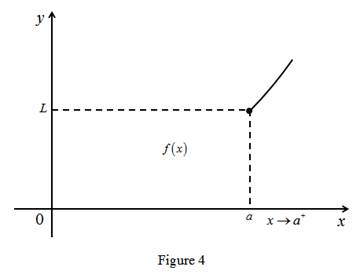(c)

To determine

To explain: The meaning of limxaf(x)=L.

Explanation

Result used:

Definition of limit:

Let f(x) be a function is defined when x approaches to p then limxpf(x)=L, if for every number ε>0 there is some δ>0  such that |f(x)L|<ε whenever 0<|xp|<δ.

Calculation:

The limit of the function limxaf(x)=L means the limit of f(x) equal to L when x approaches to a from the left, if x is closer and closer to a from the left and remains less than a then the value of f(x) also closer and closer to L.

Graph: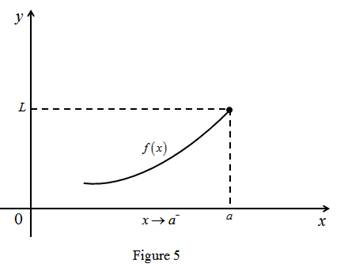(d)

To determine

To explain: The meaning of limxaf(x)=.

Explanation

Result used:

Definition of limit:

Let f(x) be a function is defined when x approaches to p then limxpf(x)=L, if for every number ε>0 there is some δ>0  such that |f(x)L|<ε whenever 0<|xp|<δ.

Calculation:

The limit of the function limxaf(x)= means the limit of f(x) is larger value when x approaches to a from the both sides. That is any M>0, f(x)>M for some x-value is sufficiently close to a.

Graph:(e)

To determine

To explain: The meaning of limxf(x)=L.

Explanation

Result used:

Definition of limit:

Let f(x) be a function is defined when x approaches to p then limxpf(x)=L, if for every number ε>0 there is some δ>0  such that |f(x)L|<ε whenever 0<|xp|<δ.

Calculation:

The limit of the function limxf(x)=L means the limit of f(x) is L when x approaches to larger value the graph get closer and closer to the line y=L.

Graph:#### The Solution to Your Study Problems

Bartleby provides explanations to thousands of textbook problems written by our experts, many with advanced degrees!

Get Started

#### Find more solutions based on key concepts